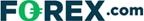# Weighted Moving Average: What It Means in Technical Trading

Last Updated:

The weighted moving average is a technical analysis indicator that reacts better to recent price changes. On this page, we discuss how it is calculated and how to interpret the data with the help of an example chart.

## What Is the Weighted Moving Average?

The weighted moving average (WMA) places more importance on recent price moves. Therefore, the WMA reacts more quickly to price changes than the regular simple moving average.

## How to Calculate WMA

Here is a basic example (three-period) of how the weighted moving average is calculated:

• Prices for the past three days have been \$5, \$4, and \$8.
• Since there are three periods, the most recent day (\$8) gets a weight of 3, the second most recent day (\$4) receives a weight of 2, and the first day (\$5) receives a weight of just 1.
• The calculation is as follows: [(3 x \$8) + (2 x \$4) + (1 x \$5)] / 6 = \$6.17

Now compare the weighted moving average value of 6.17 to the simple moving average calculation of 5.67. Note how the large price increase that occurred on the most recent day was better reflected in the weighted moving average calculation.

## WMA Chart Example

This chart of Wal-Mart stock illustrates the visual difference between a 10-day weighted moving average and a 10-day simple moving average.

Potential buy and sell signals for the weighted moving average indicator are discussed in-depth on the simple moving average indicator page.

## Where to Trade Commodities Using Technical Analysis

If you’re interested in trading using technical analysis indicators like the weighted moving average, have a look at our reviews of these regulated brokers available in .

CFDs are complex instruments and come with a high risk of losing money rapidly due to leverage. Between 74%-89% of retail investor accounts lose money when trading CFDs. You should consider whether you can afford to take the high risk of losing your money.

For information on the other moving average indicators, you can check out the following pages:

For all the basics on how to trade commodities, see our introduction to commodity trading.

Also, see our guides on forex, crypto, and options brokers to find out which technical charting tools online brokerages offer their clients.

## FAQ

### What Does Weighted Moving Average Forecast?

The weighted moving average (WMA) measures market momentum by assigning more weight to recent data than to past data.

This is done by giving each data point a different weighting factor based on its recency. The resultant moving average follows prices more closely than a simple moving average for the same period.

### Plus500 is not available in the US

Legitimate CFD brokers, like Plus500, cannot accept US clients by law## US traders welcome at these brokers:

### Cryoptocurrencies:• Trade 14+ major crypto coins
• Includes Bitcoin, Ethereum & Ripple
• Super simple setup